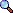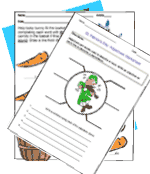# Five Ways To Make Teaching and Learning Math Fun

### Math Addition Starters Workbook | Math Worksheet MakerSubtraction Starter Pack Worksheets range from: single and double digit subtraction, identifying differences, nontraditional subtraction arrangement, identifying even and odds, introduction to greater than / less than, and subtraction word problems .Multiplication Starter Pack Single-triple digit multiplication, multiplication activities, mixed and same number multiplication, tic tac toe math, chain multiplication, multiplication box math, multiplication word problems.

 Five Ways To Make Teaching and Learning Math Fun 1. Shopping games. 2. Create math projects that relate locally. 3. Write word problems that really hit home. 4. Get them moving. Engage students with activties that require movement. 5. Use technology.Need 30,000 More Worksheets?50,000+ WorksheetsLesson PlansTeacher PrintablesClass ActivitiesMS Office TemplatesWeb Quests